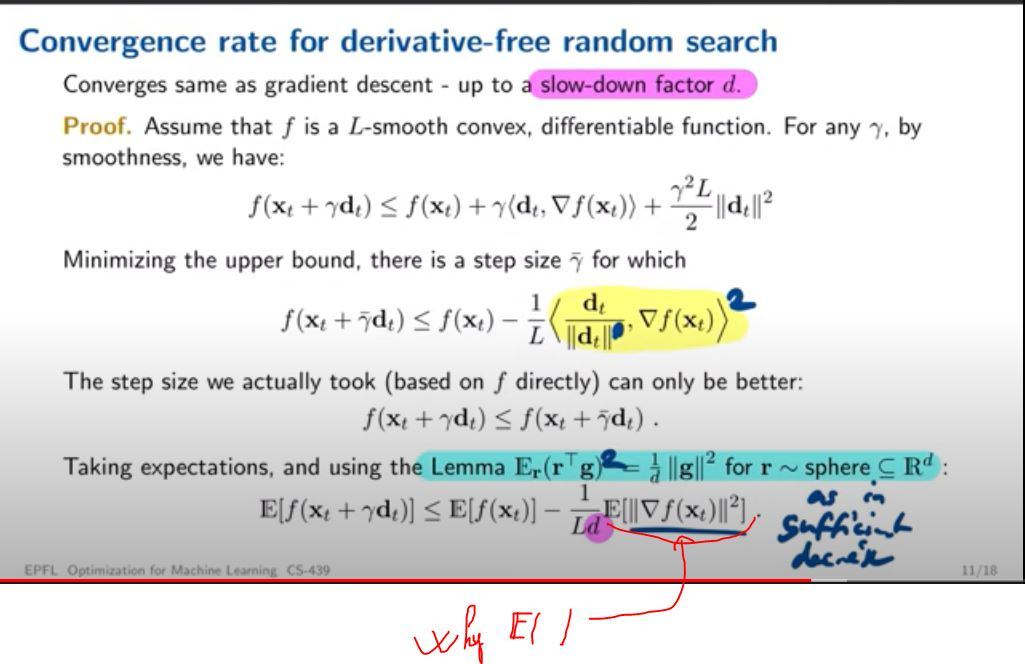### Lecture 10 slide 11

Hello,
In the slide 11 of the Lecture 10. I don't understand why we have the expectation of the gradient of f, the lemma doesn't say that.I'm also curious to know the name of this lemma, (I'm wondering if the sphere of r should have a radius ? or if there is a specific distribution)

Thank you

Top comment

yes you're right the very last expectation can be removed, as the gradient which serves as g here is deterministic indeed

the lemma works for any fixed direction g, it is not in any way related to gradients. for the sphere though, you're right it holds for radius 1 only (unit sphere). similar results exist for the Gaussian distribution (with unit variance) in space, but here we used the unit sphere

Thanks for answering the 2nd point.
For the first question. What I mean is why we have the expectation (as I highlighted on the image), we should have the gradient only without expectation ?

Top comment

yes you're right the very last expectation can be removed, as the gradient which serves as g here is deterministic indeed